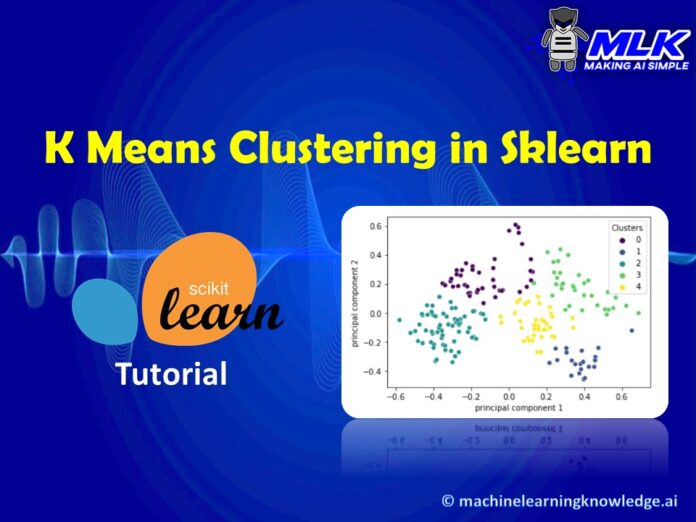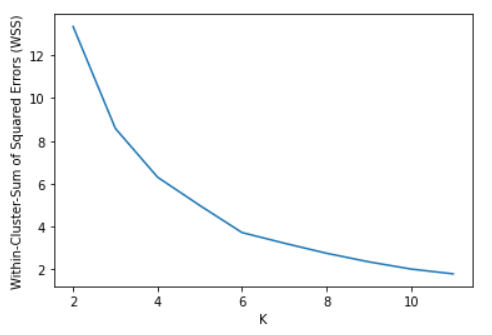# Tutorial for K Means Clustering in Python SklearnIn :
Out:
Annual Income (k\$) Spending Score (1-100)
0 0.000000 0.387755
1 0.000000 0.816327
2 0.008197 0.051020
3 0.008197 0.775510
4 0.016393 0.397959

### Applying Kmeans with 2 Clusters (K=2)

Let us see how to apply K-Means in Sklearn to group the dataset into 2 clusters (0 and 1). The output shows the cluster (0th or 1st) corresponding to the data points in the dataset.

Continuing with our example, we calculate the WCSS for K=2 to k=12 and calculate the WCSS in each iteration.

In :

```K=range(2,12)
wss = []

for k in K:
kmeans=cluster.KMeans(n_clusters=k)
kmeans=kmeans.fit(df_scale)
wss_iter = kmeans.inertia_
wss.append(wss_iter)```

Let us now plot the WCSS vs K cluster graph. It can be seen below that there is an elbow bend at K=5 i.e. it is the point after which WCSS does not diminish much with the increase in value of K.

In :

```import sklearn.cluster as cluster
import sklearn.metrics as metrics
for i in range(2,13):
labels=cluster.KMeans(n_clusters=i,random_state=200).fit(df_scale).labels_
print ("Silhouette score for k(clusters) = "+str(i)+" is "
+str(metrics.silhouette_score(df_scale,labels,metric="euclidean",sample_size=1000,random_state=200)))```
Out:
```Silhouette score for k(clusters) = 2 is 0.33340205479521
Silhouette score for k(clusters) = 3 is 0.4514909309424474
Silhouette score for k(clusters) = 4 is 0.49620078745146784
Silhouette score for k(clusters) = 5 is 0.5594854531227246
Silhouette score for k(clusters) = 6 is 0.5380652777999084
Silhouette score for k(clusters) = 7 is 0.43787929453711455
Silhouette score for k(clusters) = 8 is 0.43074523601514214
Silhouette score for k(clusters) = 9 is 0.4421331695270676
Silhouette score for k(clusters) = 10 is 0.44563877983976935
Silhouette score for k(clusters) = 11 is 0.44293254541345917
Silhouette score for k(clusters) = 12 is 0.4427512711673661```

### Applying Kmeans with 5 Clusters (K=5)

Now that we have identified that the optimum value of K is 5
```scaler = MinMaxScaler()

scale = scaler.fit_transform(df[['Age','Annual Income (k\$)','Spending Score (1-100)']])

df_scale = pd.DataFrame(scale, columns = ['Age','Annual Income (k\$)','Spending Score (1-100)']);

Out:

In :

Let us again use the elbow method with Within-Cluster-Sum of Squared Error (WCSS) to determine the optimum value of K. From the graph it looks like there is a bend between 5 and 6.
In :
Out:### Applying Kmeans with 5 Clusters (K=5)

K Mean clustering is applied with SKlearn KMeans() by passing the value of k=5

```pca_df['Clusters'] = kmeans.labels_

sns.scatterplot(x="principal component 1", y="principal component 2",hue = 'Clusters',  data=pca_df,palette='viridis')```